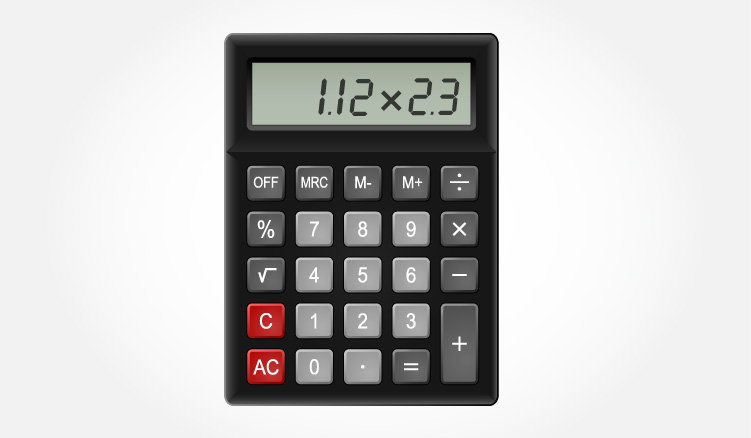C Program to Multiply two Floating Point Numbers

In this program, user is asked to enter two numbers (floating point numbers). Then, the product of those two numbers is stored in a variable and displayed on the screen.To understand this example, you should have the knowledge of following C programming topics:

Program to Multiply Two Numbers

#include <stdio.h>
int main()
{
double firstNumber, secondNumber, product;
printf("Enter two numbers: ");

// Stores two floating point numbers in variable firstNumber and secondNumber respectively
scanf("%lf %lf", &firstNumber, &secondNumber);

// Performs multiplication and stores the result in variable productOfTwoNumbers
product = firstNumber * secondNumber;

// Result up to 2 decimal point is displayed using %.2lf
printf("Product = %.2lf", product);

return 0;
}

Output

Enter two numbers: 2.4
1.12
Product = 2.69

In this program, user is asked to enter two numbers. These two numbers entered by the user is stored in variable firstNumber and secondNumber respectively. This is done using scanf() function.

Then, the product of firstNumber and secondNumber is evaluated and the result is stored in variable productOfTwoNumbers.

Finally, the productOfTwoNumbers is displayed on the screen using printf() function.

Notice that, the result is round to second decimal place using %.2lf conversion character.